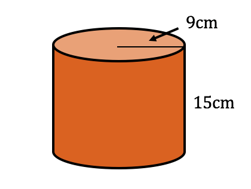PRIMARY

3 - 6

INTERMEDIATE

7 - 8

SECONDARY

9 - 11

Home

# Volume of a Cylinder

Theory & Practice Questions.

[Formula]:

V = πr²h

## Example

Find the volume of this cylinder correct to two decimal places.

##Solution

Substitute r = 9 and h = 15

V = πr²h

= π * (9)² * 15

=1215π

=3817.04cm³